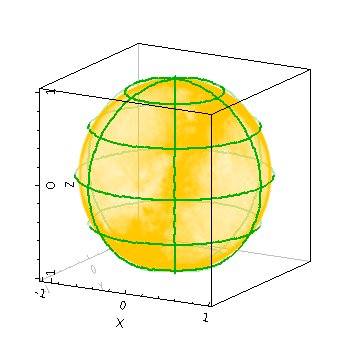Next Previous Up Contents
Next: yerror
Up: Layer Types
Previous: line3d

#### 8.3.46 `spheregrid`

Plots a spherical grid around the origin of a 3-d plot. The radius of the sphere can be configured explicitly, otherwise a suitable default value (that should make at least some of the grid visible) will be chosen.

Usage Overview:

```   layerN=spheregrid radiusN=<number> gridcolorN=<rrggbb>|red|blue|...
thickN=<pixels> nlonN=<int-value> nlatN=<int-value>
```

All the parameters listed here affect only the relevant layer, identified by the suffix `N`.

Example:```   stilts plot2sphere legend=false xpix=350 ypix=350
layer1=mark in1=tgas_source.fits lon1=ra lat1=dec r1=1
layer2=spheregrid gridcolor2=green thick2=2
```

`gridcolorN = <rrggbb>|red|blue|...`       (Color)
The color of the spherical grid, given by name or as a hexadecimal RGB value.

The standard plotting colour names are `red`, `blue`, `green`, `grey`, `magenta`, `cyan`, `orange`, `pink`, `yellow`, `black`, `light_grey`, `white`. However, many other common colour names (too many to list here) are also understood. The list currently contains those colour names understood by most web browsers, from `AliceBlue` to `YellowGreen`, listed e.g. in the Extended color keywords section of the CSS3 standard.

Alternatively, a six-digit hexadecimal number RRGGBB may be supplied, optionally prefixed by "`#`" or "`0x`", giving red, green and blue intensities, e.g. "`ff00ff`", "`#ff00ff`" or "`0xff00ff`" for magenta.

[Default: `grey`]

`nlatN = <int-value>`       (Integer)
Number of latitude lines to plot both above and below the equator in the spherical grid. A value of zero plots just the equator, and negative values plot no parallels at all.

[Default: `2`]

`nlonN = <int-value>`       (Integer)
Number of longitude great circles to plot in the spherical grid. Since each great circle joins the poles in two hemispheres, this value is half the number of meridians to be drawn.

[Default: `3`]

`radiusN = <number>`       (Double)
Defines the radius of the spherical grid that is drawn around the origin. Positive values give the radius in data units, negative values provide a multiplier for the default radius which is chosen on the basis of the currently visible volume.

[Default: `-1`]

`thickN = <pixels>`       (Integer)
Thickness of plotted line in pixels.

[Default: `1`]

Next Previous Up Contents
Next: yerror
Up: Layer Types
Previous: line3d

STILTS - Starlink Tables Infrastructure Library Tool Set